## 20090228

### Astronomy clicker question: Doppler shifted absorption spectra

Astronomy 210, Spring Semester 2009
Cuesta College, San Luis Obispo, CA

Students were asked the following clicker question (Classroom Performance System, einstruction.com) at the end of their learning cycle:

Which absorption spectrum corresponds to a star moving towards the Earth? (The arrows indicate the expected wavelength values for a stationary star of the same type.)(N.b.: (E) is the "I'm lost, and don't know how to answer this" response.)

Section 30674 (pre-)
(A) : 17 students
(B) : 10 students
(C) : 1 student
(D) : 1 student
(E) : 2 students

This question was asked again after displaying the tallied results with the lack of consensus, with the following results. No comments were made by the instructor, in order to see if students were going to be able to discuss and determine the correct answer among themselves.

Section 30674 (post-)
(A) : 23 students
(B) : 7 students
(C) : 0 students
(D) : 0 students
(E) : 1 student

For a source moving towards away from an observer, all absorption wavelengths are redshifted ("stretched") to slightly longer values. Note that the rightmost absorption line in spectra (B) is not even shifted at all (likewise, the leftmost absorption line in spectra (D) is unshifted as well).

Pre- to post- peer-interaction gains:
pre-interaction correct = 55%
post-interaction correct = 74%
Hake (normalized) gain <g> = 43%

## 20090227

### Astronomy clicker question: photon energy requirement

Astronomy 210, Spring Semester 2009
Cuesta College, San Luis Obispo, CA

Students were asked the following clicker question (Classroom Performance System, einstruction.com) at the end of their learning cycle:

A photon with slightly more energy than is required for an electron to move between energy levels 1 -> 2 would:
(A) be absorbed, causing the electron to move between energy levels 1 -> 2.
(B) not be absorbed, such that the electron stays in energy level 1.
(C) (Not enough information is given to answer this.)
(D) (I'm lost, and don't know how to answer this.)

Section 30674 (pre-)
(A) : 17 students
(B) : 10 students
(C) : 4 students
(D) : 0 students

This question was asked again after displaying the tallied results with the lack of consensus, with the following results. No comments were made by the instructor, in order to see if students were going to be able to discuss and determine the correct answer among themselves.

Section 30674 (post-)
(A) : 12 students
(B) : 17 students
(C) : 2 students
(D) : 0 students

A photon must have exactly the right amount of energy in order to be absorbed by an electron and cause the electron to make an orbital transition. "No more, no less."

Some students persistent in believing that an electron could absorb a photon with more than enough energy required. However in the quantum world: "Exact change only."

Pre- to post- peer-interaction gains:
pre-interaction correct = 32%
post-interaction correct = 55%
Hake (normalized) gain <g> = 33%

## 20090226

### Physics clicker question: accelerating elevator force magnitudes

Physics 205A, Spring Semester 2009
Cuesta College, San Luis Obispo, CA

Cf. Giambattista/Richardson/Richardson, Physics, 2/e, Problem 4.22

Students were asked the following clicker questions (Classroom Performance System, einstruction.com) in the middle of their learning cycle:

A 2,010 kg elevator moves with an upward acceleration of 1.50 m/s^2.

Which force has the least magnitude?
(A) Net force on the elevator.
(B) Weight of the elevator.
(C) Tension force of cable on the elevator.
(D) (There is a tie.)
(E) (I'm lost, and don't know how to answer this.)

Sections 30880, 30881
(A) : 29 students
(B) : 11 students
(C) : 0 students
(D) : 0 students
(E) : 1 student
(F) : 0 students

This question was asked again after displaying the tallied results with the lack of consensus, with the following results. No comments were made by the instructor, in order to see if students were going to be able to discuss and determine the correct answer among themselves.

Sections 30880, 30881
(A) : 37 students
(B) : 6 students
(C) : 0 students
(D) : 0 students
(E) : 0 students
(F) : 0 students

The free-body diagram for the elevator is discussed in a previous post:

Physics clicker question: accelerating elevator free-body diagrams

The weight force is given by m*g = 1.97e4 N, upwards. The net force, from Newton's second law, is m*a = 3.02e3 N, upwards. Thus the magnitude of the upwards tension force must be greater than the weight force by 3.02e3 N, which must then be 2.27e4 N, making it the force with the greatest magnitude, and of these three choices, net force has the least magnitude.

Pre- to post- peer-interaction gains:
pre-interaction correct = 71%
post-interaction correct = 86%
Hake, or normalized gain = 52%

## 20090225

### Physics clicker question: accelerating elevator free-body diagrams

Physics 205A, Spring Semester 2009
Cuesta College, San Luis Obispo, CA

Cf. Giambattista/Richardson/Richardson, Physics, 2/e, Problem 4.22

Students were asked the following clicker questions (Classroom Performance System, einstruction.com) in the middle of their learning cycle:

A 2,010 kg elevator moves with an upward acceleration of 1.50 m/s^2.

Which free-body diagram shows the tension and weight forces acting on the elevator, and the resulting net force?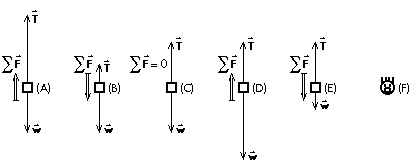(N.b.: (F) is the "I'm lost, and don't know how to answer this" response.)

Sections 30880, 30881
(A) : 25 students
(B) : 7 students
(C) : 6 students
(D) : 2 students
(E) : 3 students
(F) : 0 students

This question was asked again after displaying the tallied results with the lack of consensus, with the following results. No comments were made by the instructor, in order to see if students were going to be able to discuss and determine the correct answer among themselves.

Sections 30880, 30881
(A) : 39 students
(B) : 0 students
(C) : 0 students
(D) : 1 student
(E) : 0 students
(F) : 0 students

The elevator is accelerating upwards, thus net force must point upwards as well, from Newton's second law. Thus FDBs (B) and (E) are eliminated for having downwards net forces, and FBD (C) is eliminated for having a zero net force. FBD (D) has the downwards weight force more than the upwards tension force, which is not consistent with its upwards net force, and thus be eliminated for being internally inconsistent. (Similarly, FBD (E) is also "impossible.")

FBD (A) correctly shows an upwards net force, and the corresponding upwards net force as having a greater magnitude than the downwards weight force.

Pre- to post- peer-interaction gains:
pre-interaction correct = 58%
post-interaction correct = 98%
Hake, or normalized gain = 94%

## 20090224

### Astronomy quiz question: accurately describing planetary motion

Astronomy 210 Quiz 2, Spring Semester 2009
Cuesta College, San Luis Obispo, CA

__________ solved the problem of accurately describing planetary motion.
(A) Copernicus.
(B) Tycho.
(C) Kepler.
(D) Galileo.

While Copernicus placed the sun at the center of the planetary orbits, these orbits were circular, and thus could neither accurately describe nor predict planetary motion. Kepler analyzed Tycho's observations to come with empirical laws that accurately described planetary motion along elliptical orbits.

Section 30676
(A) : 13 students
(B) : 5 students
(C) : 39 students
(D) : 10 students

"Success level": 59% (including partial credit for multiple-choice)
Discrimination index (Aubrecht & Aubrecht, 1983): 0.77

## 20090223

### Astronomy quiz question: total solar eclipse, seen from the Moon

Astronomy 210 Quiz 2, Spring Semester 2009
Cuesta College, San Luis Obispo, CA

[4.0 points.] If observers in San Luis Obispo, CA, were observing a total solar eclipse, what would be seen by an observer on the side of the Moon facing Earth?
(A) A partial solar eclipse.
(B) An annular solar eclipse.
(C) The Sun, with no part of it being blocked by Earth.
(D) Circular umbral and penumbral shadow zones passing across the surface of Earth.

Section 30676
(A) : 7 students
(B) : 5 students
(C) : 6 students
(D) : 45 students

The Moon will be between Earth and the Sun during a total solar eclipse, and an observer on the side of the Moon facing the Earth will watch the Earth passing through the shadow of the Moon.

"Difficulty level": 73% (including partial credit for multiple-choice)
Discrimination index (Aubrecht & Aubrecht, 1983): 0.59

## 20090222

### Astronomy quiz question: total solar eclipse phase

Astronomy 210 Quiz 2, Spring Semester 2009
Cuesta College, San Luis Obispo, CA

[4.0 points.] What phase is the Moon in during a total solar eclipse?
(A) New.
(B) Full.
(C) (The phase depends on whether the total solar eclipse occurs at sunrise, midnight, or sunset.)
(D) (The Moon does not have a phase during a total solar eclipse.)

Section 30676
(A) : 29 students
(B) : 31 students
(C) : 1 student
(D) : 1 student
(No response: 1 student)

The Moon will be between Earth and the Sun, and thus will be new during a total solar eclipse.

"Difficulty level": 50% (including partial credit for multiple-choice)
Discrimination index (Aubrecht & Aubrecht, 1983): 0.49

## 20090221

### Astronomy quiz question: parallax of Tycho's supernova

Astronomy 210 Quiz 2, Spring Semester 2009
Cuesta College, San Luis Obispo, CA

[4.0 points.] Tycho could not observe any parallax for a new star (a supernova) in 1572, proving that:
(A) the heavens cannot be perfect and unchanging.
(B) Earth cannot be the center of motion in the universe.
(C) retrograde motion is caused by planets in different orbits around the sun.
(D) the sun cannot be the center of motion in the universe.

Section 30674
(A) : 13 students
(B) : 19 students
(C) : 5 students
(D) : 1 student

Since there was no apparent parallax for the supernova, it was deemed to be located out way past the earthly sphere, in the heavens, which no longer could be though of as static in nature.

"Difficulty level": 38% (including partial credit for multiple-choice)
Discrimination index (Aubrecht & Aubrecht, 1983): 0.64

## 20090220

### Astronomy quiz question: midnight Venus?

Astronomy 210 Quiz 2, Spring Semester 2009
Cuesta College, San Luis Obispo, CA

[4.0 points.] Why is it not possible for Venus to be seen at midnight (12 AM) for an observer in San Luis Obispo, CA?
(A) Venus' orbit around the Sun is smaller than Earth's orbit.
(B) Venus revolves faster around the Sun than does Earth.
(C) Venus does not undergo retrograde motion.
(D) Venus' orbit around the Sun is not circular as Earth's orbit.

Section 30674
(A) : 23 students
(B) : 7 students
(C) : 6 students
(D) : 2 students

Venus cannot be behind Earth, with respect to the Sun, and thus cannot be seen by an observer at midnight (at least, for San Luis Obispo, CA).

"Difficulty level": 64% (including partial credit for multiple-choice)
Discrimination index (Aubrecht & Aubrecht, 1983): 0.82

## 20090219

### Astronomy quiz question: total lunar eclipse phase

Astronomy 210 Quiz 2, Spring Semester 2009
Cuesta College, San Luis Obispo, CA

[4.0 points.] What phase is the Moon in during a total lunar eclipse?
(A) New.
(B) Full.
(C) (The phase depends on whether the total lunar eclipse occurs at sunrise, midnight, or sunset.)
(D) (The Moon does not have a phase during a total lunar eclipse.)

Section 30674
(A) : 10 students
(B) : 21 students
(C) : 4 students
(D) : 2 students
(No response: 1 student)

The Moon will be behind Earth with respect to the Sun, and thus will be full during a total lunar eclipse.

"Difficulty level": 58% (including partial credit for multiple-choice)
Discrimination index (Aubrecht & Aubrecht, 1983): 0.64

## 20090218

### Overheard: atmospheric turburlence

Astronomy 210, Spring Semester 2009
Cuesta College, San Luis Obispo, CA

Announcing the question for students to ponder while reading ahead for the next lecture:

Instructor: "Here's your 'question to ponder': 'What makes stars twinkle?'"

Student: "Because of that song children sing."

Instructor: "Then make them stop singing it!"

## 20090217

### Physics clicker question: average acceleration from initial and final velocity vectors

Physics 205A, Spring Semester 2009
Cuesta College, San Luis Obispo, CA

Cf. Giambattista/Richardson/Richardson, Physics, 2/e, Problem 3.41

Students were asked the following clicker questions (Classroom Performance System, einstruction.com) at the start of their learning cycle:Suppose a car travels one-quarter around a circle in a time of 1.0 s at a constant speed of 31.4 m/s, as shown at right.

Which red vector is delta(v) = v_f - v_i for this 1.0 s time interval?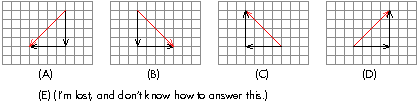Sections 30880, 30881
(A) : 18 students
(B) : 20 students
(C) : 1 student
(D) : 2 students
(E) : 1 student

This question was asked again after displaying the tallied results with the lack of consensus, with the following results. No comments were made by the instructor, in order to see if students were going to be able to discuss and determine the correct answer among themselves.

Sections 30880, 30881
(A) : 10 students
(B) : 32 students
(C) : 0 students
(D) : 2 students
(E) : 0 students

The vector operation v_f - v_i can be interpreted as tail-to-head vector addition if the direction of v_i is reversed, such that delta(v) = v_f + (-v_i).

Pre- to post- peer-interaction gains:
pre-interaction correct = 48%
post-interaction correct = 73%
Hake, or normalized gain = 48%

Subsequently, students were asked to submit numerical answers to the following clicker question:

Note: a_av = delta(v)/delta(t) = (v_f - v_i)/delta(t).

The average acceleration magnitude of the car for this one-quarter around trip is _____ m/s^2.

Sections 30880, 30881
"-5" : 1 student
"-1.75" : 1 student
"-1" : 2 students
"0" : 19 students
"2" : 3 students
"4" : 1 student
"7.85" : 1 student
"8" : 2 students
"31.4" : 9 students
"44.4" : 2 students

Correct response: 44.4 m/s^2.

Due to too few students being able to answer this correctly, this question was not asked again for peer discussion. Instead, the instructor facilitated a whole-class discussion to determine why students chose certain answers.

"0" m/s^2 was chosen because the speed of the car was constant. However, the direction is changing, and this by itself requires a non-zero acceleration.

"2," "4," "7.85" and "8" m/s^2 were probably chosen due to reading the graph scales figuratively (even though no such scale was given).

"-1" m/s^2 was chosen because it was the "slope" of the a_av vector, thus confusing vector operations with kinematic graphs.

The length of the resultant delta(v) vector is 44.4 m/s (from applying the Pythagorean theorem), and thus a_av = delta(v)/delta(t) = 44.4 m/s^2.

## 20090216

### Physics quiz question: final velocity of falling object

Physics 205A Quiz 2, Spring Semester 2009
Cuesta College, San Luis Obispo, CA

Cf. Giambattista/Richardson/Richardson, Physics, 2/e, Problem 2.45

[3.0 points.] Consider a ball that is thrown vertically upwards with an initial speed of 4.20 m/s from the roof of a building that is 52.7 m tall. Neglect air resistance. With what velocity does the ball strike the ground?
(A) –32.4 m/s.
(B) –32.1 m/s.
(C) –31.9 m/s.
(D) –22.6 m/s.

Since time is not explicitly given, the most direct approach is to solve for v_f,y using:

(v_fy)^2 - (v_iy)^2 = 2*a_y*delta(y),

where v_iy = +4.20 m/s, a_y = -9.80 m/s^2, and delta(y) = -52.7 m.

Response (B) is using v_iy = 0 (this was the most common error). Response (C) is the result of a sign error in solving for v_fy as sqrt(-v_iy^2 + 2*a_y*delta(y)). Response (D) is the result of v_iy*delta(y)/a_y.

Student responses
Sections 30880, 30881
(A) : 28 students
(B) : 10 students
(C) : 6 students
(D) : 4 students

"Difficulty level": 60%
Discrimination index (Aubrecht & Aubrecht, 1983): 0.87

## 20090215

### Physics quiz question: displacement from velocity versus time graph

Physics 205A Quiz 2, Spring Semester 2009
Cuesta College, San Luis Obispo, CA

Cf. Giambattista/Richardson/Richardson, Physics, 2/e, Problem 2.11[3.0 points.] Consider the v_x(t) graph of an object moving along a straight line, as shown at right. How far does the object move between t = 0 s and t = 8.0 s?
(A) 18 m.
(B) 25 m.
(C) 28 m.
(D) 35 m.

The displacement (straight-line distance traveled) by object is the area under a v_x(t) graph, which in this case is a trapezoid.

Response (C) is the distance traveled by the object if it had uniformly increased its speed from rest to 7.0 m/s for the entire 8.0 s; response (A) is the distance traveled by the object if it had uniformly increased its speed from rest to 7.0 m/s from 3.0 s to 8.0 s. Response (D) is the difference between the distance traveled by the object had it continuously traveling at 7.0 m/s for the entire 8.0 s, and the correct response (B).

Student responses
Sections 30880, 30881
(A) : 2 students
(B) : 39 students
(C) : 6 students
(D) : 1 student

"Difficulty level": 81%
Discrimination index (Aubrecht & Aubrecht, 1983): 0.27

## 20090214

### Physics quiz question: accelerating car

Physics 205A Quiz 2, Spring Semester 2009
Cuesta College, San Luis Obispo, CA

Cf. Giambattista/Richardson/Richardson, Physics, 2/e, Comprehensive Problem 2.57(a)

[3.0 points.] Consider a car traveling in the +x direction that accelerates uniformly from a speed of 15 m/s to 22 m/s in a time of 12 s. How far did the car travel during this time?
(A) 84 m.
(B) 180 m.
(C) 222 m.
(D) 264 m.

The displacement (straight-line distance traveled) by the car is the area under a v_x(t) graph, which in this case is a trapezoid, with heights v_ix = 15 m/s and v_if = 22 m/s, and base delta(t) = 12 s, such that the total area can either be expressed as (1/2)*(v_ix + v_if)*delta(t), or "rectangle and triangle" v_ix*delta(t) + (1/2)*(v_fx - v_ix)*delta(t).

Response (D) is the distance traveled by the car if it had a speed of 22 m/s for the entire 12 s, while response (B) is the distance traveled by the car if it had a speed of 15 m/s for the entire 12 s. Response (A) is the difference between response (D) and the correct response (C).

Student responses
Sections 30880, 30881
(A) : 8 students
(B) : 0 students
(C) : 38 students
(D) : 2 students

"Difficulty level": 79%
Discrimination index (Aubrecht & Aubrecht, 1983): 0.40

## 20090213

### Learning never ends...090122-1070814
http://flickr.com/photos/waiferx/3229797006/

Learning never ends...but it may not necessarily begin.

## 20090212

### Physics clicker question: free fall acceleration

Physics 205A, Spring Semester 2009
Cuesta College, San Luis Obispo, CA

Cf. Giambattista/Richardson/Richardson, Physics, 2/e, Multiple-Choice Question 2.1

Students were asked the following clicker questions (Classroom Performance System, einstruction.com) in the middle of their learning cycle (after being asked about the acceleration while the ball is traveling downwards, and while traveling upwards):

A ball is thrown and released such that it initially travels upwards. While it is momentarily at its highest point, its acceleration is __________. (Up = +y direction; neglect air resistance ("free fall").)
(A) +9.80 m/s^2.
(B) 0 m/s^2.
(C) –9.80 m/s^2.
(D) (More than one of the above choices.)
(E) (None of the above choices.)
(F) (I'm lost, and don't know how to answer this.)

Sections 30880, 30881
(A) : 0 students
(B) : 30 students
(C) : 13 students
(D) : 0 students
(E) : 0 students
(F) : 0 students

A whole-class discussion on velocity versus time graphs for objects in free fall immediately followed the tallying of results. All v_y(t) graphs for free fall may have different initial v_i,y values, but would all have the same negative slopes, and thus acceleration values of -9.80 m/s^2.

## 20090211

### Physics 205A laboratory, Cuesta College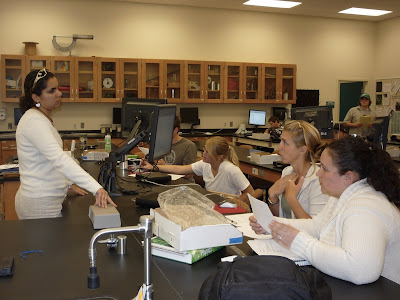090130-1070954
http://flickr.com/photos/waiferx/3264478669/

Lab instructor Ms. Mitra Farahmand answers questions from students Courtney and Alexis in Physics 205A lab in the newly renovated 2100 building.090130-1070955
http://flickr.com/photos/waiferx/3264481041/

Layout of student computer stations in Room 2101 of the newly renovated 2100 building at Cuesta College.

## 20090210

### Astronomy clicker question: planet orbit position

Astronomy 210, Spring Semester 2009
Cuesta College, San Luis Obispo, CA

Students were asked the following clicker question (Classroom Performance System, einstruction.com) at the end of their learning cycle:

Consider the view of the eastern horizon shown below, as seen from San Luis Obispo, CA at a certain date/time. Carefully note the positions of the Sun, and an unknown planet.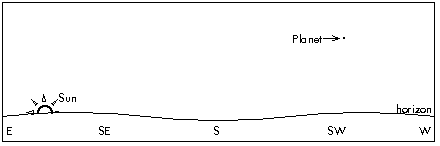Which of planetary positions (A)-(E) shown below corresponds to the planet shown above? (This drawing is not to scale, and orbits have been simplified as circles instead of ellipses.)(F) = (I'm lost and don't know how to answer this.)

Section 30676 (pre-)
(A) : 6 students
(B) : 1 student
(C) : 25 students
(D) : 7 students
(E) : 2 students
(F) : 4 students

This question was asked again after displaying the tallied results with the lack of consensus, with the following results. No comments were made by the instructor, in order to see if students were going to be able to discuss and determine the correct answer among themselves.

Section 30676 (post-)
(A) : 0 students
(B) : 0 students
(C) : 43 students
(D) : 1 student
(E) : 0 students
(F) : 1 student

Since the Sun is in the east, this is sunrise (6 AM), and the planet is found near the west horizon, away from the Sun.

Pre- to post- peer-interaction gains:
pre-interaction correct = 56%
post-interaction correct = 96%
Hake (normalized) gain <g> = 90%

Section 30674 (pre-)
(A) : 2 students
(B) : 0 students
(C) : 14 students
(D) : 3 students
(E) : 10 students
(F) : 2 students

Section 30674
(A) : 0 students
(B) : 0 students
(C) : 31 students
(D) : 0 students
(E) : 0 students
(F) : 0 students

Pre- to post- peer-interaction gains:
pre-interaction correct = 45%
post-interaction correct = 100%
Hake (normalized) gain <g> = 100%

## 20090209

### Astronomy quiz question: moorise to moonset time

Astronomy 210 Quiz 1, Spring Semester 2009
Cuesta College, San Luis Obispo, CA

[4.0 points.] In general, approximately how much time elapses between moonrise to moonset?
(A) An hour.
(B) 12 hours.
(C) 24 hours.
(D) 28 days.

Section 30676
(A) : 0 students
(B) : 62 students
(C) : 1 student
(D) : 1 student

The Moon takes approximately 12 hours to rise and set (with variations due to its motion along its orbit around the Earth, and its position with regards to the ecliptic due to the tilt of its orbit).

"Difficulty level": 94% (including partial credit for multiple-choice)
Discrimination index (Aubrecht & Aubrecht, 1983): 0.11

## 20090208

### Astronomy quiz question: time for constellation zenith-to-set motion

Astronomy 210 Quiz 1, Spring Semester 2009
Cuesta College, San Luis Obispo, CA

[4.0 points.] Cygnus is at the zenith (directly overhead), as seen by an observer at 12 AM (midnight) in San Luis Obispo, CA. (Ignore the fact that your starwheel does not include daylight savings time.) How many hours will pass until this constellation completely sets below the horizon?
(A) 3 hours.
(B) 6 hours.
(C) 9 hours.
(D) (This constellation is circumpolar, and will never set.)

Section 30676
(A) : 2 students
(B) : 20 students
(C) : 42 students
(D) : 4 students

Using a starwheel for the lower 48 states (San Luis Obispo, CA), stars that are at the zenith will set 9 hours later.

"Difficulty level": 66% (including partial credit for multiple-choice)
Discrimination index (Aubrecht & Aubrecht, 1983): 1.00

*Corrected from a comment by a student.

## 20090207

### Astronomy quiz question: daylight hours

Astronomy 210 Quiz 1, Spring Semester 2009
Cuesta College, San Luis Obispo, CA

[Version 1]

Consider the position of the Sun at some point on its path across the sky on a certain day, as seen by an observer in San Luis Obispo, CA.[4.0 points.] On this date, approximately how much time will the Sun be above the horizon, from rise to set?
(A) 10 hours.
(B) 12 hours.
(C) 14 hours.
(D) 30 days.

Section 30674
(A) : 13 students
(B) : 6 students
(C) : 1 student
(D) : 0 students

For the equinoxes of March or September, the Sun would rise from the east, and set in the west, spending 12 hours above the horizon. If the Sun rises south of east, and sets south of west, it will spend less than 12 hours above the horizon.

[Version 2]

Consider the position of the Sun at some point on its path across the sky on a certain day, as seen by an observer in San Luis Obispo, CA.[4.0 points.] On this date, approximately how much time will the Sun be above the horizon, from rise to set?
(A) 10 hours.
(B) 12 hours.
(C) 14 hours.
(D) 30 days.

Section 30674
(A) : 7 students
(B) : 6 students
(C) : 4 students
(D) : 0 students

For the equinoxes of March or September, the Sun would rise from the east, and set in the west, spending 12 hours above the horizon. If the Sun rises north of east, and sets north of west, it will spend more than 12 hours above the horizon.

"Difficulty level": 49% (including partial credit for multiple-choice)
Discrimination index (Aubrecht & Aubrecht, 1983): 0.90

## 20090206

### Astronomy quiz question: highest point in sky for east-rising stars

Astronomy 210 Quiz 1, Spring Semester 2009
Cuesta College, San Luis Obispo, CA

[4.0 points.] Virgo is just above the east horizon, as seen by an observer at 11 PM in San Luis Obispo, CA. (Ignore the fact that your starwheel does not include daylight savings time.) Where will this constellation be at its highest point in the sky?
(A) Somewhere between the north horizon and the north celestial pole.
(B) Somewhere between the north celestial pole and the zenith.
(C) Somewhere very close to the zenith.
(D) Somewhere between the south horizon and the zenith.

Section 30674
(A) : 1 student
(B) : 6 students
(C) : 4 students
(D) : 26 students

Using a starwheel for the lower 48 states (San Luis Obispo, CA), stars that rise directly in the east will set directly in the west, but will not pass directly overhead, at the zenith. Instead, these stars will cross the meridian between the south horizon and the zenith for their highest point in the sky.

"Difficulty level": 71% (including partial credit for multiple-choice)
Discrimination index (Aubrecht & Aubrecht, 1983): 0.64

## 20090205

### Physics clicker question: crossed graphs, part 2

Physics 205A, Spring Semester 2009
Cuesta College, San Luis Obispo, CA

Cf. Knight, Five Easy Lessons: Strategies for Successful Physics Teaching, p. 85

Students were asked the following clicker questions (Classroom Performance System, einstruction.com) in the middle of their learning cycle: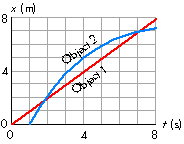Consider the x(t) graph of two objects moving along a straight line for t = 0 to t = 8 s, as shown at right. Do object 1 and object 2 have the same speed?
(A) Yes, at t = 2 s.
(B) Yes, at t = 7 s.
(C) Yes, sometime between t = 2 s and 7 s.
(D) (More than one of the above choices.)
(E) No.
(F) (I'm lost, and don't know how to answer this.)

Sections 30880, 30881
(A) : 0 students
(B) : 0 students
(C) : 27 students
(D) : 0 students
(E) : 15 students
(F) : 0 students

This question was asked again after displaying the tallied results with the lack of consensus, with the following results. No comments were made by the instructor, in order to see if students were going to be able to discuss and determine the correct answer among themselves.

Sections 30880, 30881
(A) : 0 students
(B) : 0 students
(C) : 36 students
(D) : 0 students
(E) : 6 students
(F) : 0 students

The tangent slope of a position versus time graph is the (instantaneous) velocity. Since object 2 has a tangent slope that is identical to the slope of object 1, then they have the same velocity, and thus the same speed between t = 2 s and 7 s.

Pre- to post- peer-interaction gains:
pre-interaction correct = 64%
post-interaction correct = 86%
Hake, or normalized gain = 60%

## 20090204

### Physics clicker question: crossed graphs, part 1

Physics 205A, Spring Semester 2009
Cuesta College, San Luis Obispo, CA

Cf. Knight, Five Easy Lessons: Strategies for Successful Physics Teaching, p. 85

Students were asked the following clicker questions (Classroom Performance System, einstruction.com) in the middle of their learning cycle:Consider the x(t) graph of two objects moving along a straight line for t = 0 to t = 8 s, as shown at right. Do object 1 and object 2 have the same speed?
(A) Yes, at t = 4 s.
(B) Yes, sometime between t = 0 and t = 4 s.
(C) Yes, sometime between t = 4 s and 8 s.
(D) (More than one of the above choices.)
(E) No.
(F) (I'm lost, and don't know how to answer this.)

Sections 30880, 30881
(A) : 10 students
(B) : 3 students
(C) : 0 students
(D) : 1 student
(E) : 27 students
(F) : 0 students

This question was asked again after displaying the tallied results with the lack of consensus, with the following results. No comments were made by the instructor, in order to see if students were going to be able to discuss and determine the correct answer among themselves.

Sections 30880, 30881
(A) : 3 students
(B) : 0 students
(C) : 0 students
(D) : 0 students
(E) : 39 students
(F) : 0 students

The slope of a position versus time graph is the velocity. Since these two objects have different slopes, they have different velocities, and thus, different speeds.

Pre- to post- peer-interaction gains:
pre-interaction correct = 66%
post-interaction correct = 93%
Hake, or normalized gain = 79%

## 20090203

### Discover your weight on Earth, the Moon, and Mars090201-1070957
http://www.flickr.com/photos/waiferx/3247179968/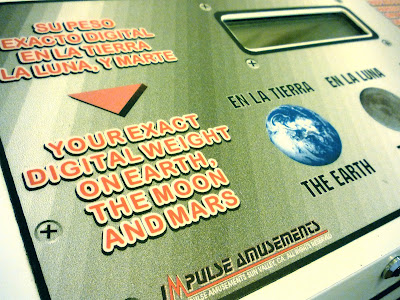090201-1070958
http://www.flickr.com/photos/waiferx/3247182114/

Discover your weight on Earth, the Moon, and Mars! Pay weight scale, Union 76 gas station, Skyline Boulevard/Avenal Cutoff Road exit at I-5, Avenal, CA.

## 20090201

### Physics quiz question: unit conversion and significant figures

Physics 205A Quiz 1, spring semester 2009
Cuesta College, San Luis Obispo, CA

Cf. Giambattista/Richardson/Richardson, Physics, 2/e, Problem 1.31

Evaluate the following calculation, and express the answer in units of m^3, using an appropriate number of significant figures and/or decimal places.

(3.2 m) * (1.242 cm) * (6.0*10^3 m) = ?

(A) 2*10^2 m^3.
(B) 2.4*10^2 m^3.
(C) 2*10^4 m^3.
(D) 2.4*10^4 m^3.

1.242 cm must be converted to 1.242*10^-2 m. Also the first and third lengths are expressed to only two significant figures, which will limit the final answer to two significant figures.

Response (A) does not have the correct number of significant figures. Response (D) has the correct number of significant figures, but cm was not converted to m. Response (C) has both an incorrect number of significant figures, and no cm to m conversion.

Student responses
Sections 30880, 30881
(A) : 3 students
(B) : 34 students
(C) : 0 students
(D) : 13 students

"Difficulty level": 68%
Discrimination index (Aubrecht & Aubrecht, 1983): 0.50